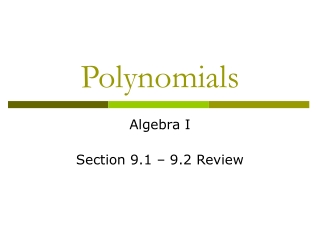DownloadDownload PresentationPolynomials

# Polynomials

Télécharger la présentation## Polynomials

- - - - - - - - - - - - - - - - - - - - - - - - - - - E N D - - - - - - - - - - - - - - - - - - - - - - - - - - -
##### Presentation Transcript

1. Polynomials Algebra I Section 9.1 – 9.2 Review

2. Polynomials Polynomial: A monomial or a sum of monomials. Monomial: 1 term 4xy2 Binomial: 2 terms 5x - 1 Trinomial: 3 terms 3x2 – 2x + 1 Polynomial: 4 terms or more

3. Degree of a Polynomial To find the degree of a polynomial (if it is a: monomial), add the exponents on the variables contained in each term. Note: If more than one term has the same degree, arrange the terms by which single base has the highest degree. Examples: 1. 8y4 2. 3a 3. -2xy2z3 4. 7

4. Degree of a Polynomial Constant = 0 Linear = 1 Quadratic = 2 Cubic = 3

5. Classifying Polynomials –3x5 + 9x3 + 7 Trinomial Degree 5 5x4y6 Monomial Degree 10 –8xy + 15x Binomial Degree 1 6x6 + 2x3 + 3x2 Trinomial Degree 6

6. Standard Form of a Polynomial Place the terms of the polynomial in order from the least degree to greatest degree. Example: Put the trinomial below in standard form. 9x3 + 7 – 3x5

7. Polynomial Standard Form Practice 3x2 – 6x2y2 + 4x3 -x4 + 2xy + 7xy4 -9rx + r5x 15km – 13m3 + 10k3

8. Adding & Subtracting Polynomials (3x2 – 4x + 8) + (2x – 7x2 – 5) Combine “like-terms” and put solution in standard form.

9. Practice Adding & Subtracting Polynomials 6n2 – 4 (+) -2n2 + 9 (4x + 5xy + 3y) – (3y + 6x + 8xy)

10. Methods for Multiplying Polynomials by Polynomials 3 Methods: Distribute FOIL Box

11. Multiplying Polynomials Using Distribution Multiplying Monomials by Polynomials: Use the distributive property. Remember the Rule of Multiplying Numbers with Exponents (add the exponents) Examples: -2x2(3x2 – 7x + 10)

12. Practice Multiplying Polynomials Using Distribution 4(3d2 + 5d) 5x3(x2 – 2x + 6) 6x(2x2 – 4x) – (x + 2)

13. FOIL Method FOIL: only works when multiplying a binomial times a binomial. First – 1st terms in each parenthesis Outer – 1st term in 1st parenthesis times last term in 2nd parenthesis Inner – Last term in 1st parenthesis times 1st term in 2nd parenthesis Last – Last term in 1st parenthesis times the last term in 2nd parenthesis

14. Using FOIL (3x + 5)(x + 6) First Outer Inner Last __________ + _________ + _________ + __________ FOI L

15. Practice Using FOIL (x + 5)(x + 6) (5m + 2)(7m + 3) (8b – 3)(-2b +6) (9p + 6)(6p – 2)

16. Box Method Box Method works with all types of polynomials. The size of the box depends on how many terms are in the polynomials. Example: binomial x trinomial = 2 x 3 box

17. How to use the Box • Put each factor on the outside of a box. • Multiply to fill the box • Combine like-terms (add) to empty the boxes • Be sure to simplify your answer.

18. How to Use the Box Method (x + 2)(3x2 + 2x + 1)

19. Practice Using the Box Method (k + 4)(7k2 + 2k – 9) (2x2 – 9x + 11)(2x + 1)

20. Polynomial Word Problems The length of a pool is (2x2 + 3x) and the width is (x – 2). Find the area of the pool.

21. Polynomial Word Problems Kenny wants to build a sidewalk around a rectangular garden that is 3 feet wide. If the width of the garden is 2x and the length is 3x + 1, find the area of the sidewalk. 3x + 1 2x 3’